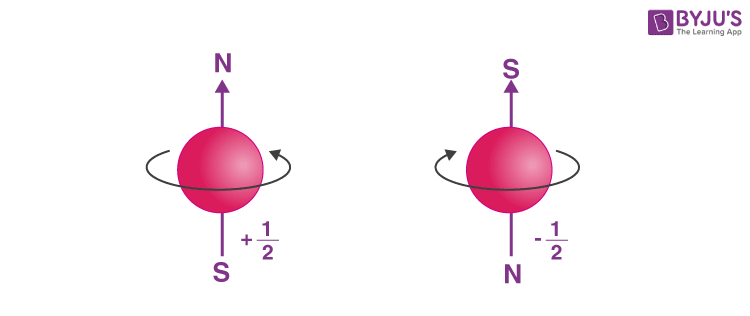# Frequently asked Questions from Quantum Numbers in JEE

Quantum numbers are actually a set of four numbers which helps in determining the complete information about the location of electrons in an atom. This is a very fundamental topic as the entire chemistry lies on the basis of electrons and their participation in a reaction. JEE gives high weight to this topic. Questions asked in this topic are easy and less time-consuming.

## Important Questions For Quantum Numbers

Question 1: What are the important topics in quantum numbers that need to be focused in order to crack JEE?

Answer: According to JEE syllabus the significant topics in quantum numbers are:

• Heisenberg’s uncertainty principle
• Quantum mechanical model of the atom
• Quantum number – principle, angular, magnetic and spin
• Size and shapes of orbitals
• Electronic configuration and rules governing the filling of electrons in orbitals
• Stability of half-filled and fully filled orbitals.

Question 2: How to determine the four set of quantum numbers?

Answer: In order to find the four set of quantum numbers we need to follow these steps:

1. Finding the value of principle quantum number: It denotes the main shell in which the electron resides. Represented by n, where n = 1, 2, 3,….
1. Find the azimuthal or angular momentum quantum number (l): It represents the number of sub shells present in a main shell. These subsidiary orbits within a shell take the values from 0, 1, 2, 3, 4,…..For a given value of n, the possible values of l varies from 0 to (n – 1). For s orbital, l=0; for p orbital, l=1; and so on.
1. Determining the magnetic quantum number (m): An electron generates an electric field due to its angular motion. This electric field produces a magnetic field. The values of ‘m’ depends on the value of l, which is the angular quantum number, m can take all integral values between –l to +l including 0.
1. Determining the spin quantum number (s): Similar to earth which not only revolves around the sun but also spins about its own axis, an electron in an atom not only revolves around the nucleus but also spins about its own axis. An electron can spin either in clockwise or anti-clockwise direction, therefore the spin quantum number can have two values, + ½ or – ½.Illustration 1:  What is the correct set of four quantum numbers for the valence electron of rubidium (Z = 37)?

Solution: The electronic configuration of Rb (Z = 37) is = 1s2 2s2 2p6 3s2 3p6 4s2 3d10 4p6 5s1

The principle quantum number, n = 5

Azimuthal quantum number, l = 0 (since for s orbital l = 0)

Magnetic quantum number, m = 0 (since for l = 0, there is only one value for m, that is 0)

Spin quantum number, s = + ½ or – ½ (there is only one electron which can have a clockwise or anticlockwise spin)

The correct set of quantum numbers will be: 5, 0, 0, + ½ or – ½

Question 3: Which books should be referred for quantum numbers while preparing for JEE?

Answer: Go through NCERT chemistry textbook for class 11th, this book contains all the details in a very precise way. NCERT is the most student-friendly book as it covers all the topics that are significant from the examination point of view. Practice questions by solving previous year’s JEE papers.

For more details on JEE or this topic install BYJU’S – the learning app.